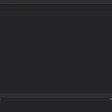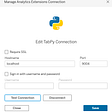# Pandas & Excel — Read, Write, Charts

Pandas is an awesome tool when it comes to manipulates data with python. Pandas also have really cool function to handle Excels files. Thought this article I will explain how to read and writes files, highlight cells and how to add charts.

# Installs and imports

Install these packages with pip

`pip install pandaspip install XlsxWriter`

And now import

`import pandas as pd`

Reading an excel file and importing it in a pandas dataframe is as simple as :

`df = pd.read_excel("file_name")`

A Dataframe is a 2-dimensional labeled data structure, it the main data structure used in pandas.

read_excel as a lot of arguments as you can see in the doc. Here are the mains ones :

• io : The file to load
• header: Index of the row where the name of the columns can be found. By default, it’s the first one.
• skiprows : number of rows to skip at the beginning of the file.
• usecols: lists of columns you want to import. If None it will import all of them.
`df = pd.read_excel(file_name, sheetname='Sheet1', header=2, skiprows=2, usecols=[0,1,2])`

# Write

To write your dataframe in an excel file

`df = pd.DataFrame({"data": [1, 2, 3, 4, 5, 6, 7]})   writer = pd.ExcelWriter(f"/tmp/file.xlsx", engine="xlsxwriter")df.to_excel(writer)writer.save()`

You can also write to a specific sheet

`df.to_excel(writer, sheet_name="Sheet 1")df2.to_excel(writer, sheet_name="Sheet 2")`

# Cells formats

Let’s adapt the formats of the cell. We want to set “Numbers“ columns with two decimals, “Percentage” should be in percentage and have the “Date” set to this format “mm/dd/yyyy”.

`df = pd.DataFrame({'Numbers':    [1, 2.244, 3.33333, 2000],                   'Percentage': [0.1, 0.33,  0.7555555555,  1],                   'Date': [datetime.datetime(2020, 10, 1, 0, 30),                            datetime.datetime(2020, 10, 1, 1, 33),                            datetime.datetime(2020, 10, 2, 2, 45),                            datetime.datetime(2020, 10, 2, 3, 30)],})#datetime_format param will set the format for all the datetime valueswriter = pd.ExcelWriter(f"/tmp/file.xlsx", engine="xlsxwriter",                        datetime_format='mm/dd/yyyy')df.to_excel(writer, sheet_name="Sheet 1")# Get xlsxwriter objectsworkbook = writer.bookworksheet = writer.sheets['Sheet 1']# Create cells formatsformat1 = workbook.add_format({'num_format': '0.00'})format2 = workbook.add_format({'num_format': '0%'})# Set the column width and format for columns Bworksheet.set_column('B:B', 20, format1)# Set the format but not the column width for columns Cworksheet.set_column('C:C', None, format2)writer.save()`

# Highlight cells

Change cells colors and background color based on a condition.

`df = pd.DataFrame({    'Data': [1, 2, 3, 4, 5, 6, 7]})writer = pd.ExcelWriter(f"/tmp/file.xlsx", engine="xlsxwriter")def color(value):    if value > 4:        color = 'red'        background = 'white'    elif 4 >= value > 2:        color = '#FFFFFF'        background = 'orange'    else:        color = 'green'        background = 'white'        # Change style of the cell     return f'color: {color}; background-color: {background}'# Apply method on all values of "Data"df = df.style.applymap(color, subset=['Data'])df.to_excel(writer)writer.save()`

We can directly add graph through the code

`df = pd.DataFrame({"data":[1, 2, 3, 4, 5, 6, 7]})   writer = pd.ExcelWriter(f"/tmp/file.xlsx", engine="xlsxwriter")df.to_excel(writer)# Get xlsxwriter objectsworkbook = writer.bookworksheet = writer.sheets['Sheet 1']# Create a 'column' chartchart = workbook.add_chart({'type': 'column'})# select the values of the series and set a name for the serieschart.add_series({    'values': '=\'Sheet 1\'!\$B\$2:\$B\$8',     "name": "My Series's Name"})# Insert the chart into the worksheet in the D2 cellworksheet.insert_chart('D2', chart)writer.save()`

Now let’s do a line plot, but instead of a string we are passing a list to values, which is more convenient to use.

There are 5 elements in the list :
- Sheet’s name as a string
- The first cell row
- The first cell column
- The last cell row
- The last cell column

`chart2 = workbook.add_chart({'type': 'line'})chart2.add_series({'values': ["Sheet 1", 1, 1, 7, 1]})worksheet.insert_chart('P2', chart2)`

/!\ indexes start at 0
Here [“Sheet 1”, 1, 1, 7, 1] will take values from B2 to B8 in Sheet 1.

You can create all your favorites charts: scatter plots, stacked bar charts, pie charts … See more here

--

--

--

## More from The Startup

Get smarter at building your thing. Follow to join The Startup’s +8 million monthly readers & +756K followers.

## Let’s Discuss About Machine Learning Algorithms..## Override date filter in Power BI## Examples of EDA for Image Analysis## KPIspy Delivers Data Analytics in Easy-to-Understand Videos for SMBs## Top features in Tabular Editor 3 to boost your Power BI development## Professional translators or Google Translate? Weighing the pros and cons of each## Visualizing Missing Data## Alexis Gomes

I work at yper. I’m a python developer, learning data science. I’ve made a www.blindfoldchesstactic.com app

## Analyzing My Personal Fitness Data## Frequently use Pandas methods/functions## Churn rate visualizations in Python## A Beginners Guide To Using Python in Tableau